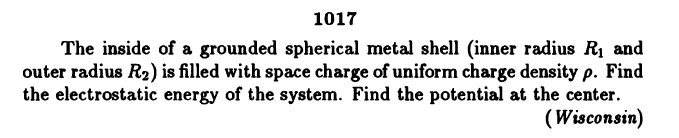# Potential inside a concentric sphere

Homework Statement:
n
Relevant Equations:
\I am rather confused how to answer this (Please focus on "find the potential at the center"):
I thought that would be a good idea try to answer this with the Poisson equation.
$$\nabla \phi = - \frac{\rho}{\epsilon}$$
So that, since the eletric field inside a hollow spherical shell is zero, the potential at the center is just equal to the potential at R1.
I found this solution
$$\phi = \sum K_{i}(\frac{\rho(R_{2}^2-R^2)}{6 \epsilon} + C(\frac{1}{R_{2}}-\frac{1}{R}))$$

But i have no idea how to go on

## Answers and Replies

haruspex
Science Advisor
Homework Helper
Gold Member
2020 Award
since the eletric field inside a hollow spherical shell is zero
Not if there are charges inside the shell.

•Herculi